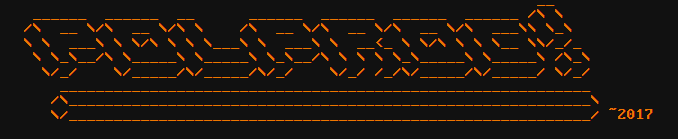Polprog dec 2018

# Easy LC circuits - part 2

In this part I will show you some simple examples of circuits that contain capacitors and inductors, to achieve a certain goal in the system.

### Simple power suppply input decoupling

Your circuit needs power to work. It can be provided from a battery, a builting power supply or an extrernal one, or even from a supercapacitor. Decoupling is used to remove noise such as sudden voltage drops and spikes from the power supply. Such noise is harmful both for analogue and digital circuits.

Supply noise in analogue circuits such as filters, buffers and the like may couple into the signal path, degrading the quality of the output. On the other side, if the power supply is not stable enough, containing rapid voltage changes or spikes may cause weird behavior of digital circuits, like locking up or resetting them.

It's a good idea to start supply filtering right at the input, like in the image belowIn that schematic snippet, J2 is the power connector. One pin goes directly to ground. The other goes through a diode which job is to protect from accidentally reversing the polarity of the power input. If you swap the polarity, D1 will not conduct. Then we have C5 which is a small 100nF capacitor that is connected between the diode output and ground. This capacitor appears as open circuit for DC current, and as a short circuit for AC current (remember the impedance formula $Z = 1/2\pi f C$?), effectively removing, or shorting-out the non-DC part of the power supply output. The VCC symbol is connected to the diode output as well (cathode of the diode, one end of the cap and the VCC symbol are electrically common).

And what about those two caps on the left between VCC and ground? They are there as a catch all group, one for each power input pin of the microcontroller that is in that schematic. Those are usually placed very close to the power rails going into chips on the real PCB, like in the images below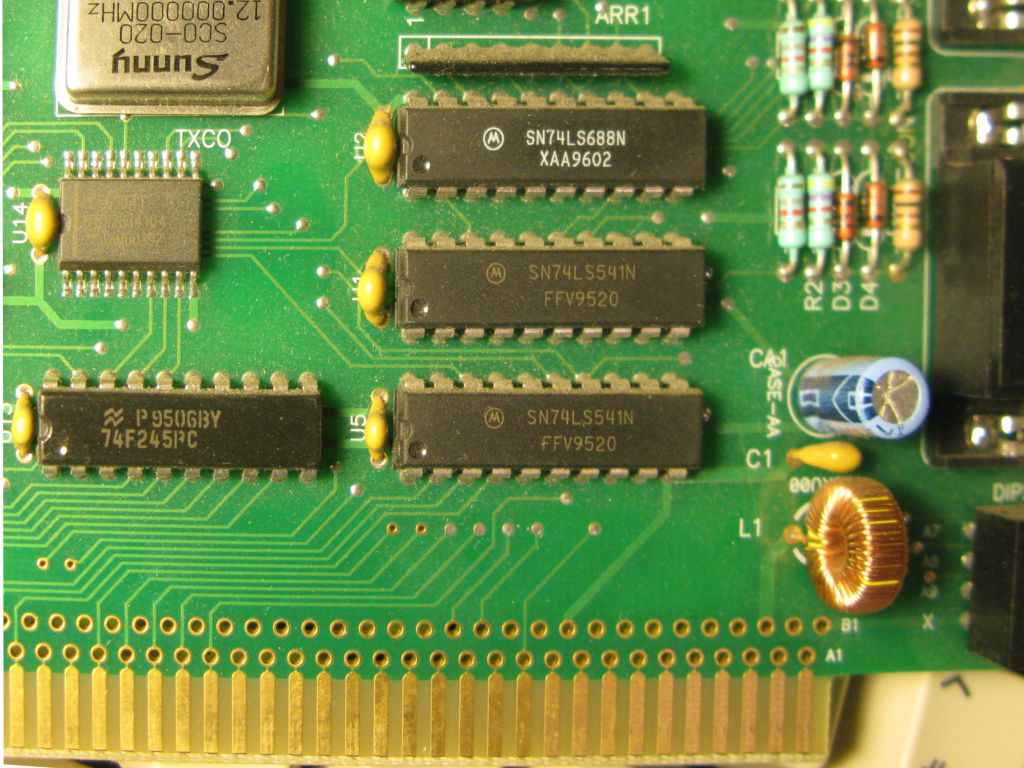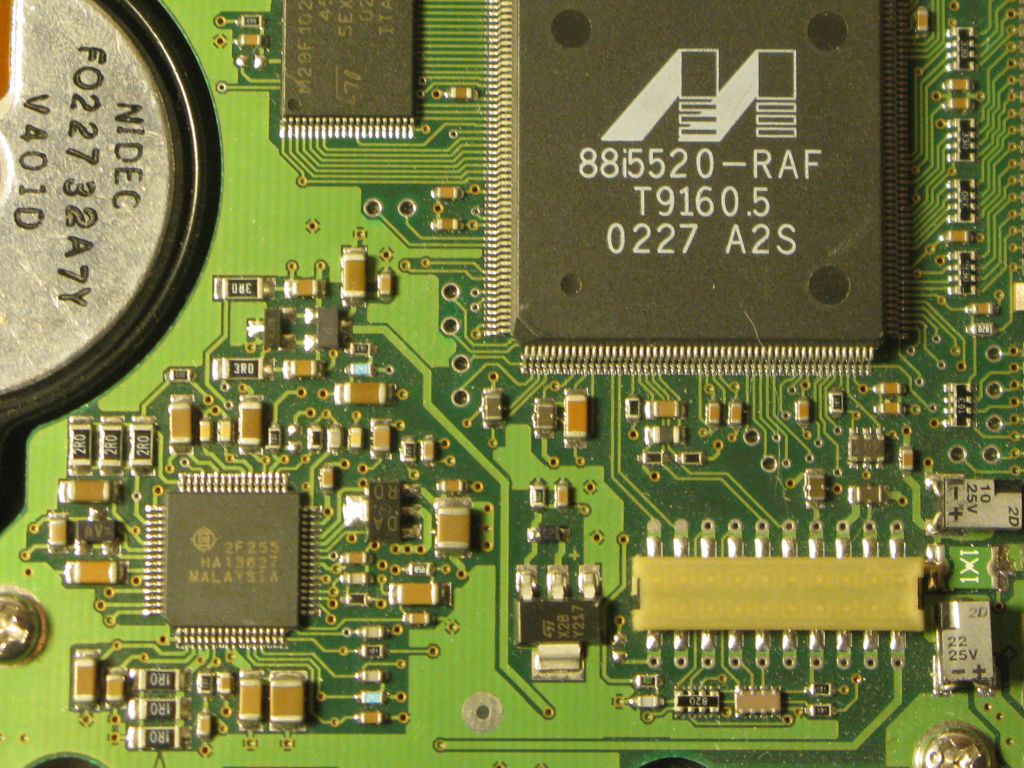Chip decoupling on two circuit boards. On the left board, there is a ceramic cap next to each of the chips. There is also a small electrolytic capacitor along with a ceramic one and an inductor. This is the power input of this ISA card.
On the right board there is a bunch of small surface-mount capacitors around every chip

Below is another example, taken from my other project that uses analogue components and some 7400-series glue logic. This one has two capacitors in paralell, a big 220 uF electrolytic capacitor (you can tell by it being polarized and the note next to it) and a small 640n foil capacitor.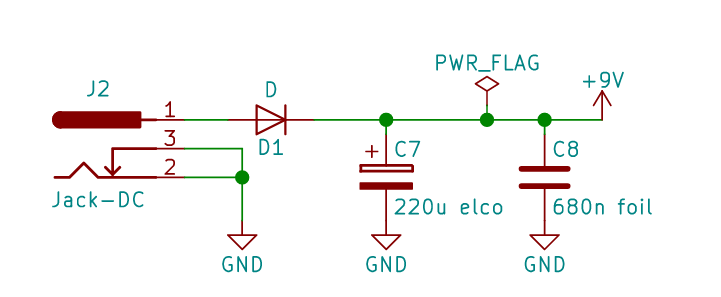Why would you put a tiny capacitor right in paralell with a huge one? After all the foil capacitor is about 500 times smaller in capacitance than the small foil one. It cannot change anything substantially, can it?

The reason for such an arrangement is that high value electrolytics often have a very high internal resistance (especially cheap ones), and are just "too slow" to compensate for voltage changes of high frequency noise. Their internal resistance also depends on the signal frequency so the higher the signal frequency, the worse the elco's efficiency in removing it. In this case C7 is responsible for "smoothing out" voltage drops due to momentary high current changes, for example, when an LED lights up on the board. The small ceramic capacitor shorts out low in amplitude but high frequency noise coming from any sources that could cause it. I remember that adding those, and others helped remove an audible hiss in the output!

Below is another example, this time using an inductor in series with a capacitor. This is a reference design of the analog-to-digital converter of a microcontroller. ADC supply voltage needs to be very stable. The inductor blocks AC current from getting into the power input, and the capacitor servers as a reservoir of energy just like in the schematics above.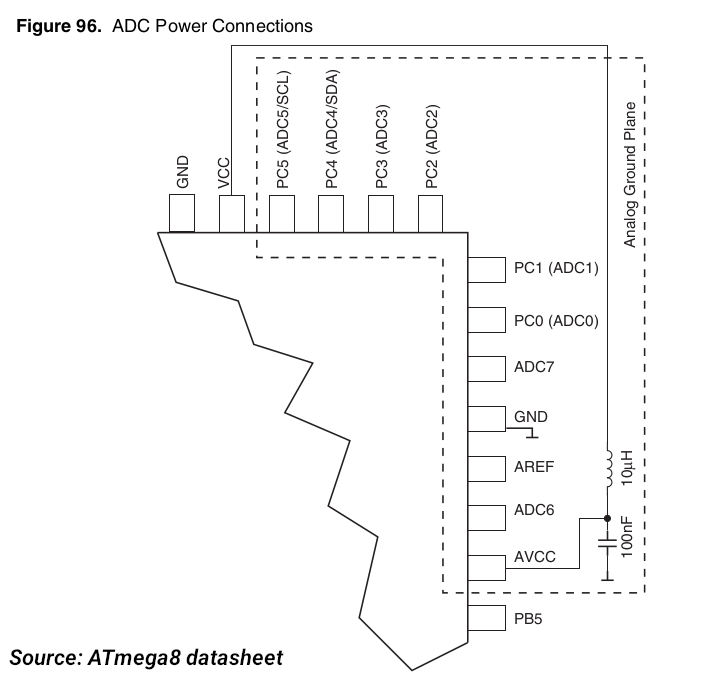### Signal coupling and filtering

Capacitors are not only used to short out AC components to ground. For example here: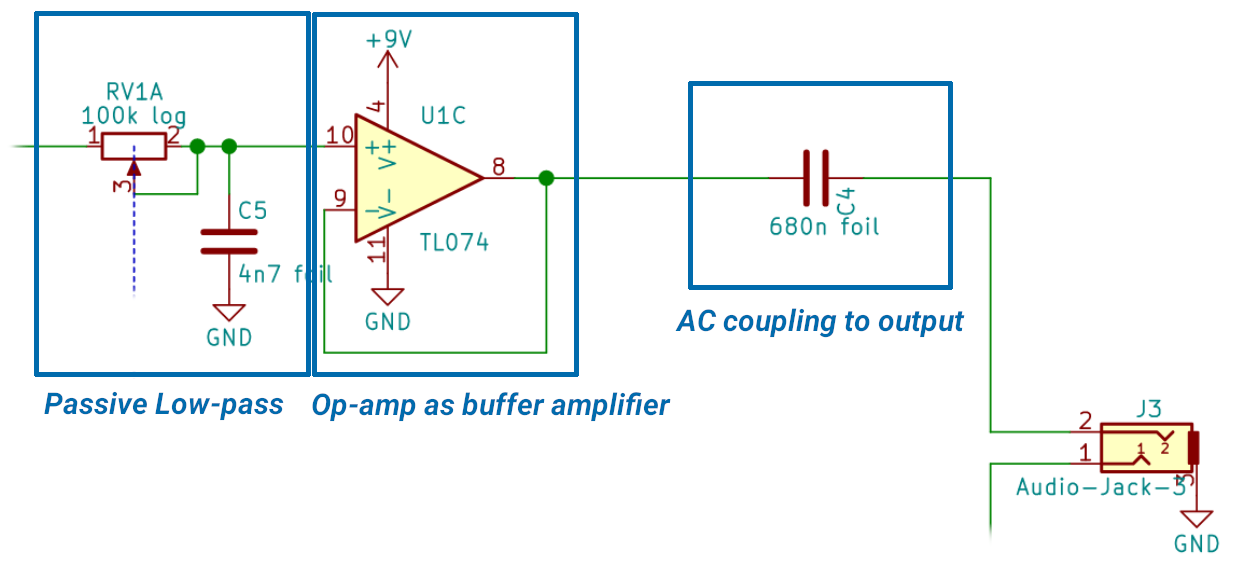This is an output stage of a circuit. The signal comes from the left, into a low-pass filter.

The filter here is a 1st order RC low pass filter. Its a voltage divider where one of the impedances (the capacitor) depends on the input signal frequency. As the input frequency rises, the capacitors impedance falls, and the divider attenuates more.

For a 1st order RC low pass filter, the cutoff frequency (a value used to describe filters), which is the frequency at which the gain is equal to -3dB, is given with the formula $$F_{3dB} = \frac{1}{2 \pi \tau} \quad \tau = RC$$ where $\tau$ is called the RC time constant

.

In this case the resistor is actually a potentiometer, with two of the pins shorted together. Not shown in the picture is a series 2k resistor. This allows us to adjust the resistance of the potentiometer from 2k to 100k + 2k = 102k. Let's run the numbers: $$F_{3dB} = \frac{1}{2 \pi R C}$$ If we set the potentiometer to its highest value, and put that into the formula we get $$R = 102 \cdot 10^3 \Omega \quad C = 4.7 \cdot 10^{-9} F\\ F_{3dB} = \frac{1}{2 \pi \cdot 102 \cdot 10^3 \cdot 4.7\cdot10^{-9}} = 331.99 Hz$$ If we set it to its lowest, we get $$R = 2 \cdot 10^3 \Omega \quad C = 4.7 \cdot 10^{-9} F\\ F_{3dB} = \frac{1}{2 \pi \cdot 2 \cdot 10^3 \cdot 4.7\cdot10^{-9}} = 16931.38 Hz$$ Which allows us to set the cutoff frequency from about 16kHz to about 330Hz.

The output of that filter goes straight to the buffer, and the buffer output is AC coupled via the capacitor to the output jack. C4 will not conduct any DC current. This is necesary to remove any DC offset from the signal.

Such a circuit can be used for example as the otuput stage of a microcontroller playing music with its DAC

Let's take a look at the counterpart of the above circuit.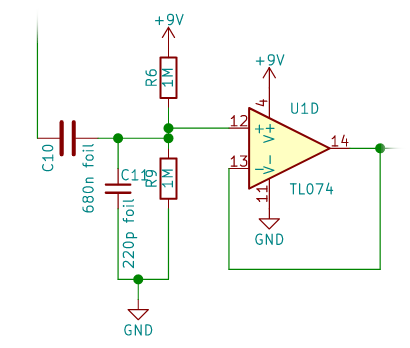The input, as previously, comes in from the left side. The signal goes through C10 which removes any DC offset and in between R9 and R6, which form a voltage divider between +9V and ground. Those two resistors are there to set a DC offset of 4.5V to the input signal, which is the middle ground between 9 and 0 volts. This is a very typical setup for a single-ended opamp supply.

The op-amps can swing their outputs only between their power supply voltages. If we connected the input signal directly to the input of the buffer it would be unable to recreate the lower half (the part of the audio signal that is below the ground voltage), instead it would just lower its output to the lowest possible value of somewhere close to zero volts.

This is why we add our own voltage bias to the signal, this way the signal's zero voltage is 4.5V, and the op-amps can handle both halves of the wave.

C10 with R6 and R5 form a different type of the filter. This is called a high-pass filter, as it attenuates low freuquency. The maths is the same than that of the low-pass filter, with the difference that it attenuates lower frequencies

The small C11 is there to remove extra high frequency noise, if any.